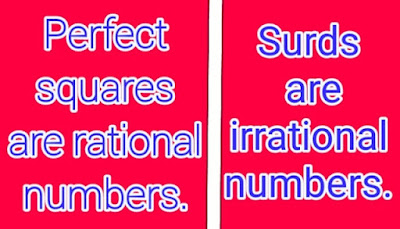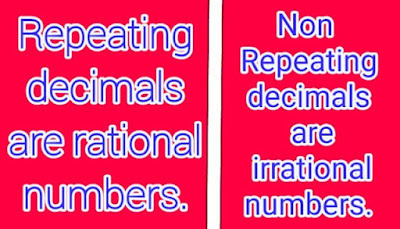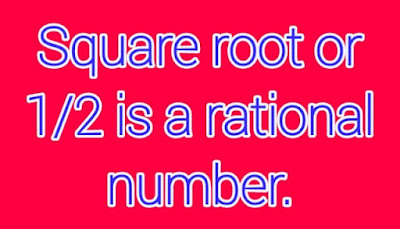### Difference Between Rational and Irrational Numbers

In Mathematics, we have to come across lots of numbers. In these numbers, there come perfect squares, surds, terminating decimals, non-terminating decimals, repeating decimals and non-repeating decimals etc. We usually divide these numbers into two categories. First category is known as rational numbers and the second category is known as irrational numbers. No doubt, to understand the difference between rational and irrational numbers is a difficult task for the students. Here, we will try to explain the difference between rational and irrational numbers with the help of examples.

# Difference Between the Definitions of Rational and Irrational Numbers

## ·        Definition of Rational numbers

In Mathematics, rational numbers are those numbers which are written in the form of p/q such that q≠0. The condition for the rational numbers is that both p and q should belong to Z and Z is a set of integers. The simplest examples of the rational numbers are given below;
·         1/9
·         10  or 10/1

## ·        Definition of Irrational Numbers

The irrational numbers are those numbers which are not written in the form of p/q. The simplest examples of the irrational numbers are given below;
·         √3
·         3/0

# Difference Between Rational and Irrational Numbers

Most of the students are not able to understand the difference between the rational and irrational numbers just with the help of their definitions. They require more detail to understand the difference between rational and irrational numbers. The key difference between them is given below;

## 1.      Perfect Squares are Rational Numbers and Surds are Irrational NumbersAll the perfect squares are rational numbers and the perfect squares are those numbers which are the squares of an integer. In other words, if we multiply an integer with the same integer, we get a perfect square. The examples of the perfect squares are √ 4, √ 49, √ 324, √ 1089 and √ 1369. After taking the square roots of these perfect squares, we get 2, 7, 18, 33 and 37 respectively. 2, 7, 18, 33 and 37 are all integers. On the other hand, all the surds are the irrational numbers and the surds are those numbers which are not the squares of an integer. In other words, these are not the multiples of an integer with itself. The examples of the surds are √2, √3 and √7. After taking the square roots of these surds, we get 1.41, 1.73 and 2.64 respectively. 1.41, 1.73 and 2.64 are not integers.

## 2.      Terminating Decimals are Rational Numbers

All the terminating decimals are rational numbers. Terminating decimals are those decimals which have finite number of digits after the decimal point. For example, 1.25, 2.34 and 6.94 are all rational numbers. On the other hand, non-terminating decimals are those numbers which have infinite number of digits after the decimal point. For example, 1.235434..., 3.4444… and 6.909090… are all non-terminating decimals. Non-terminating decimals can be rational or irrational. These are explained in the next point.

## 3.      Repeating Decimals are Rational Numbers and Non-Repeating Decimals are Irrational NumbersAll the repeating decimals are the rational numbers and the repeating decimals are those decimals whose digits repeat over and over again. The examples of the repeating decimals are .33333333, .222222 and .555555. On the other hand, all the non-repeating decimals are the irrational numbers and the non-repeating decimals are those digits which don’t repeat over and over again. The examples of the non-repeating decimals are .0435623, .3426452 and .908612.
Relevant Posts:

# Key Point

The numbers which are written without denominators are rational numbers. The examples of these kinds of numbers are 8 and 9. These numbers are written in the form of p/q as 8/1 and 9/1. The numbers whose denominators are 0 are called the irrational numbers like 8/0 and 9/0.

# Is ½ or 0.5 Rational or Irrational Number?0.5 is called the rational number because it can be written in the form of p/q like 5/10. Moreover, it is also a terminating decimal.

# Is Pi (π) Rational or Irrational Number?Pi (π)  is an irrational number. Its reason is that it gives us non-repeating decimal 3.14159……

# Is ‘0’ or ‘Zero’ Rational or Irrational?

‘0’ or ‘Zero’ is a rational number. Its reason is that ‘0’ or ‘Zero’ belongs to the set of integers and we have discussed that all the integers are rational numbers.

# Is it Possible for us to Find Irrational Numbers Between Two Rational Numbers?

It is easy for us to find irrational numbers between two rational numbers. We try to learn this concept with the help of an example. Find irrational numbers between 3 and 4. We can find the irrational numbers between these two rational numbers by following these steps;
·         First of all, we should find squares of the given numbers. In this case, the squares of 3 and 4 are 9 and 16 respectively.
·         Secondly, you should find the prime numbers between their squares. The prime numbers between 9 and 16 are 11 and 13.
·         By taking square root of these prime numbers, we get the required irrational numbers. The square roots of 11 and 13 are 3.316624… and 3.6055512… respectively. As 3.316624… and 3.6055512… are non-repeating decimals. That’s why these are irrational numbers.

# Difference Between Rational and Irrational Numbers In Tabular Form

 No. Rational Irrational 1 Surds Perfect Squares 2 Terminating ------ 3 Repeating Non-Repeating 4 √25 √23

# Key Point

You can easily express the rational numbers in the fraction form. On the other hand, you can’t express the irrational numbers in the fraction form. This is the basic difference between the rational and irrational numbers.

# Understand the Difference between Rational and Irrational Numbers with the Help of Practical Examples

After understanding the difference between rational and irrational numbers, we try to separate the rational and irrational numbers from given numbers. Separate the rational and irrational numbers from the following numbers; √5, √25, 5/4, 6/5, √36, √8, 16/3, 6/7
√5 is an irrational number because it is a surd and it is not the square of an integer with itself. √25 is a rational number because it is a square of an integer 5 with itself. 5/4 (1.25) is also a rational number because it is a terminating decimal because it has finite number of digits after the decimal point. 6/5 (1.2) is also a rational decimal because it has also finite number of digits after the decimal point. √36 is also a rational number because it is a perfect square. √8 is an irrational number because it is a surd. The answer of the fraction 16/3 is 5.33333… It means that it is a repeating decimal. As we know that repeating decimal is also a rational number. The answer of the fraction 6/7 is 0.85714… It means that it is a non-repeating decimal and we have learned that all the non-repeating decimals are irrational numbers.

# Conclusion

At the end, we are able to clearly understand the difference between rational and irrational numbers with the help of these essential points:
·         Rational numbers = Perfect squares + Terminating decimals + Repeating decimals
·         Irrational numbers = Surds + Non-repeating decimals
You just need to take an overview of a number. If it is a perfect square, terminating decimal or repeating decimal, it means that it a rational umber. On the other hand, if it is a surd or non-repeating decimal, it means that it is an irrational number.
Oldest